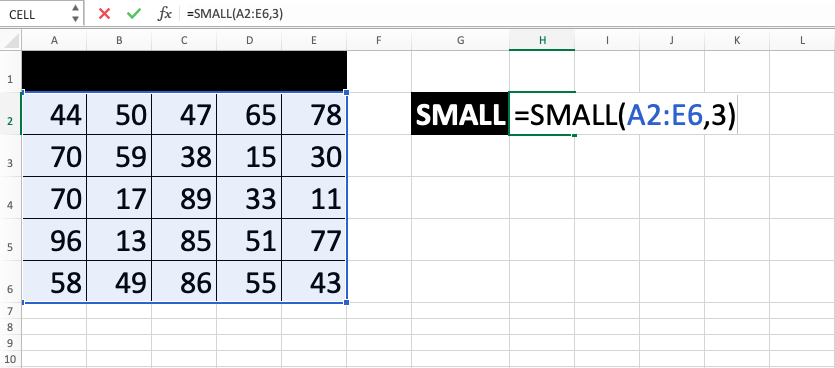SMALL Excel Formula - Compute Expert

# SMALL Excel Formula

In this tutorial, you will learn how to use the SMALL excel formula. This formula can be used if you want to find the nth smallest value from a group of numbers.

## Why do We Need to Learn SMALL Formula Excel?

In numeric data processing on a spreadsheet file, sometimes we need to find the nth smallest value from numbers. This might be needed as a material for further analysis or the final result of our data processing.

Whatever the purpose is, we should know how to do it automatically especially if there are many numbers to compare. If we don’t then we need to do it ourselves and that can be very troublesome and take too much time.

As an example of this need at work, let’s say we want to know which company branches have the smallest sales. This data might be needed so we can find the solution to improve the performance of those branches. This is so the company sales as a whole can be improved.

To identify those branches, then we need to take a look at the sales data from all branches. If there are hundreds or thousands of branches, then it will be very troublesome to look at them one by one. Because of that, we should utilize a relevant spreadsheet feature to help us if we process our data there.

For that feature, there is the SMALL excel formula to help you find the nth smallest number. You just need to write it correctly then you can get your result fast and accurately. It will be very useful to understand its use if you often do numeric data processing in a spreadsheet file.

## What is the SMALL Excel Formula?

SMALL excel formula is a formula with the usage objective to find the nth smallest value from a group of numbers. It will do a comparison process before giving you a fast and accurate result.

Generally, the inputs to use it can be explained as follows:

=SMALL(array, k)

Note:
• array = the cell range where the numbers to find the nth smallest value from are
• k = the ascending rank that you want for the result (the n from the nth smallest value)

## How to Write the SMALL Function in Excel?

The following will explain how to write the SMALL excel formula. The writing itself should be pretty easy because its inputs are quite simple.

## Using SMALL Formula in Excel

1. Type equal sign ( = ) in the cell where you want to put the result in2. Type SMALL (can be with large and small letters) and open bracket sign after =3. Input the cell range where all the numbers you want to compare to get the nth smallest value are. After that, type comma sign ( , )4. Input the ascending rank for your result/cell coordinate where the rank is5. Type close bracket sign6. Press Enter
7. The process is done!## Exercise

After learning how to write the SMALL excel formula, now is the time to do an exercise. This is so you can master its use more!

### Instruction

Find the first, second, and third smallest value of those three scores! Give your answer in the gray-colored cells with the appropriate labels!

If you instead want to find the nth largest value, then use LARGE. Learn how to use it in this Compute Expert tutorial!

Get updated excel info from Compute Expert by registering your email. It's free!